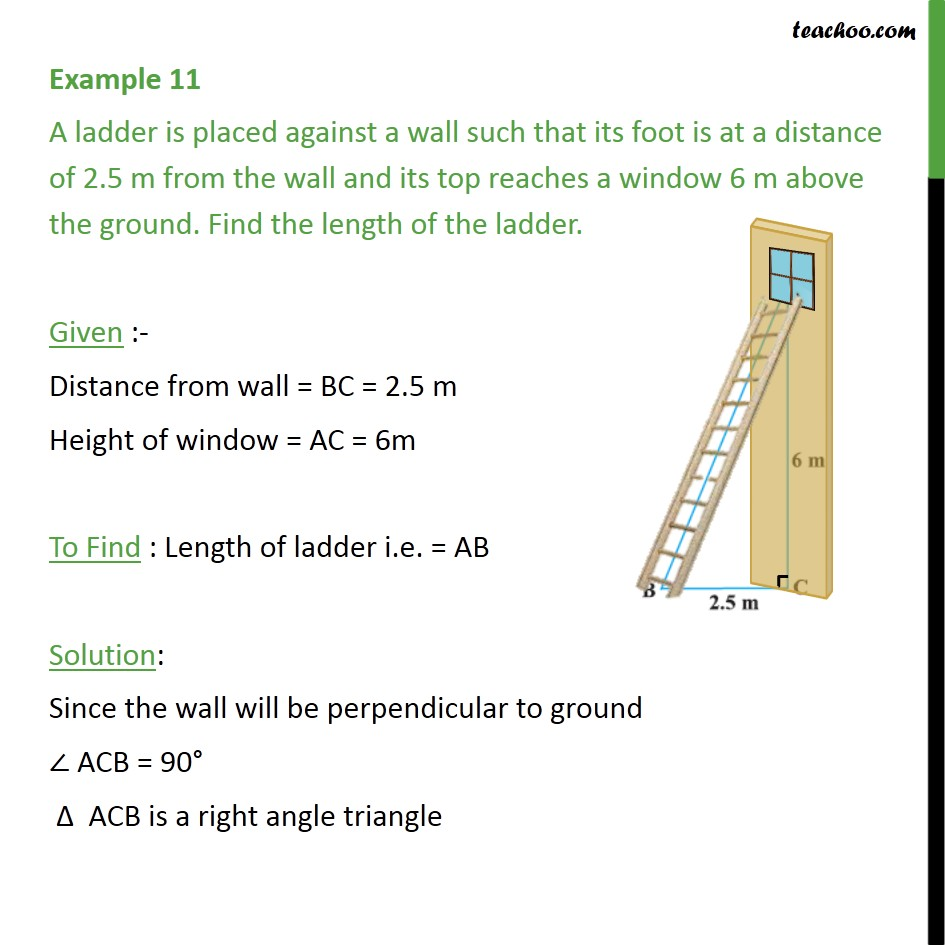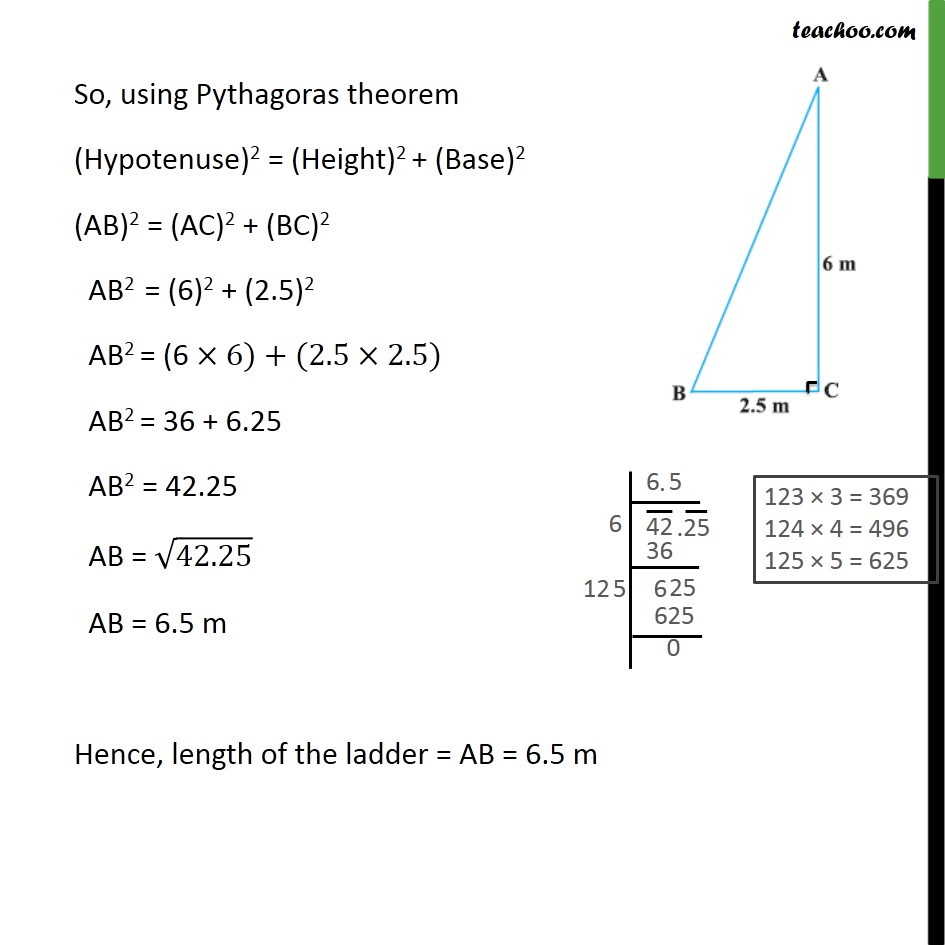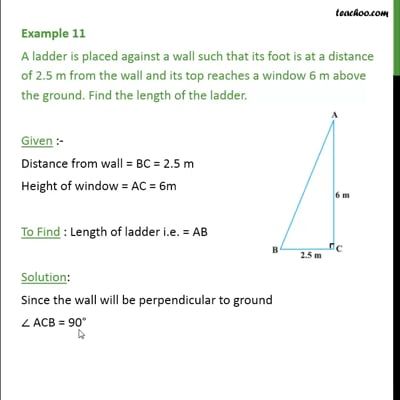Examples

Chapter 6 Class 10 Triangles (Term 1)
Serial order wiseThis video is only available for Teachoo black users

### Transcript

Example 11 A ladder is placed against a wall such that its foot is at a distance of 2.5 m from the wall and its top reaches a window 6 m above the ground. Find the length of the ladder. Given :- Distance from wall = BC = 2.5 m Height of window = AC = 6m To Find : Length of ladder i.e. = AB Solution: Since the wall will be perpendicular to ground ∠ ACB = 90° Δ ACB is a right angle triangle So, using Pythagoras theorem (Hypotenuse)2 = (Height)2 + (Base)2 (AB)2 = (AC)2 + (BC)2 AB2 = (6)2 + (2.5)2 AB2 = (6 ×6)+(2.5×2.5) AB2 = 36 + 6.25 AB2 = 42.25 AB = √42.25 AB = 6.5 m Hence, length of the ladder = AB = 6.5 m